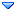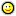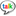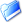GSI Forum
GSI Helmholtzzentrum für Schwerionenforschung

Home » PANDA » PandaRoot » Analysis » True vertex position for secondary decay vertices
True vertex position for secondary decay vertices Mon, 12 January 2015 21:31Ajay Kumar Messages: 102Registered: December 2011 Location: indore continuous participantFrom: 106.66.5*
Dear All,

I am trying to find true vertex position for Lambda0->p pi- and Lambdabar->pbar pi+. I have four track from the simulation and used PndMCTrack to find the true vertex position of Lambda and Lambdabar particles. I am using GetStartVertex() to find the vertex of lambda from its decay particle as below.

if(mctrack->GetSecondMotherID()==1){
if (mc_pid==2212)
{
vtx_x= mctrack->GetStartVertex().X ();
vtx_y= mctrack->GetStartVertex().Y();
vtx_z= mctrack->GetStartVertex().Z();
// r = sqrt(pow(vtx_x,2)+pow(vtx_y,2));
// countT1++;
//rzp-> Fill(vtx_z,r);
// if (vtx_z< 40)
// countT2++;

}
}
Is It giving the true vertex position of Lambda or not?
If not then how I should go for it?

Thanking you

AJAY KUMAR
Research scholar
Indian Institute of Technology Indore,IndoreTrue vertex position for secondary decay vertices By: Ajay Kumar on Mon, 12 January 2015 21:31Re: True vertex position for secondary decay vertices By: Klaus Götzen on Tue, 13 January 2015 07:25Re: True vertex position for secondary decay vertices By: Ajay Kumar on Tue, 13 January 2015 12:32Re: True vertex position for secondary decay vertices By: Klaus Götzen on Tue, 13 January 2015 15:28